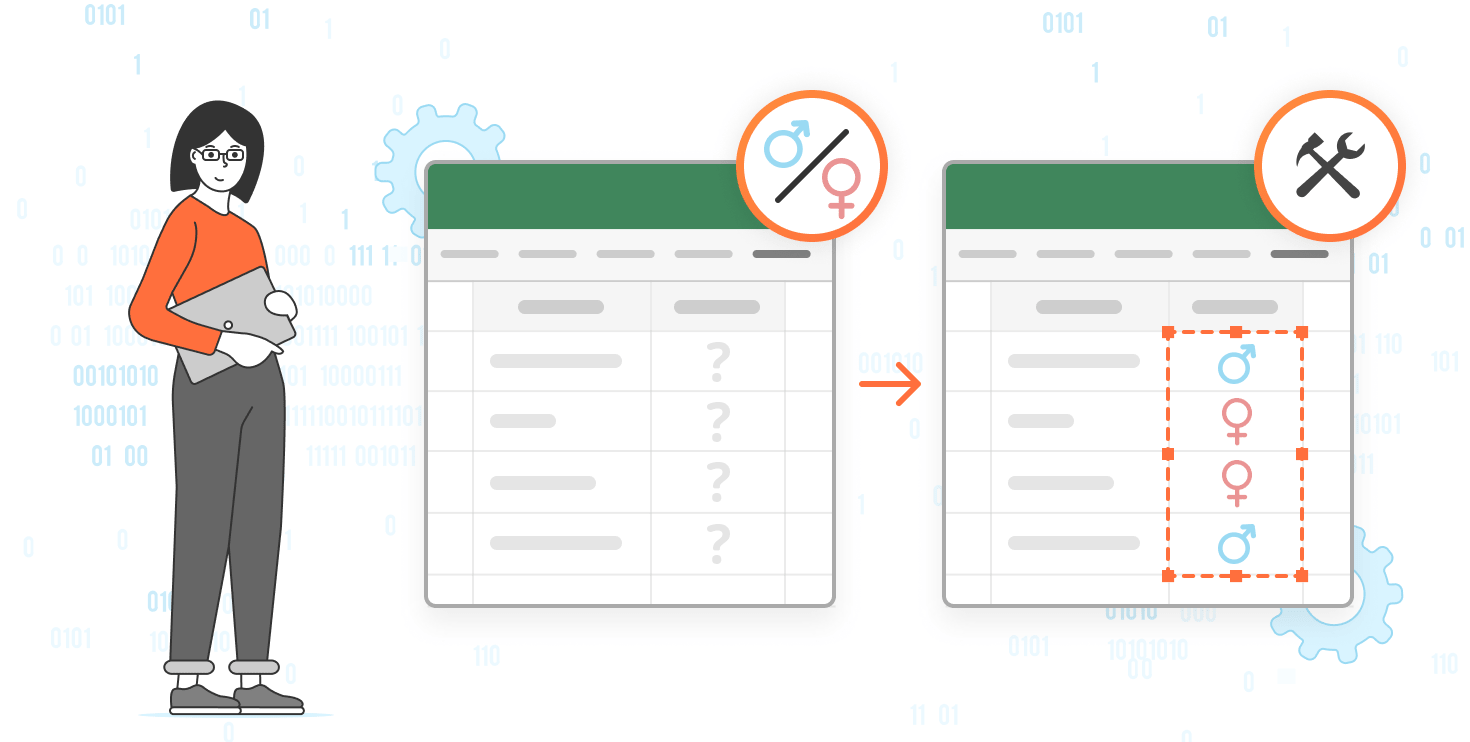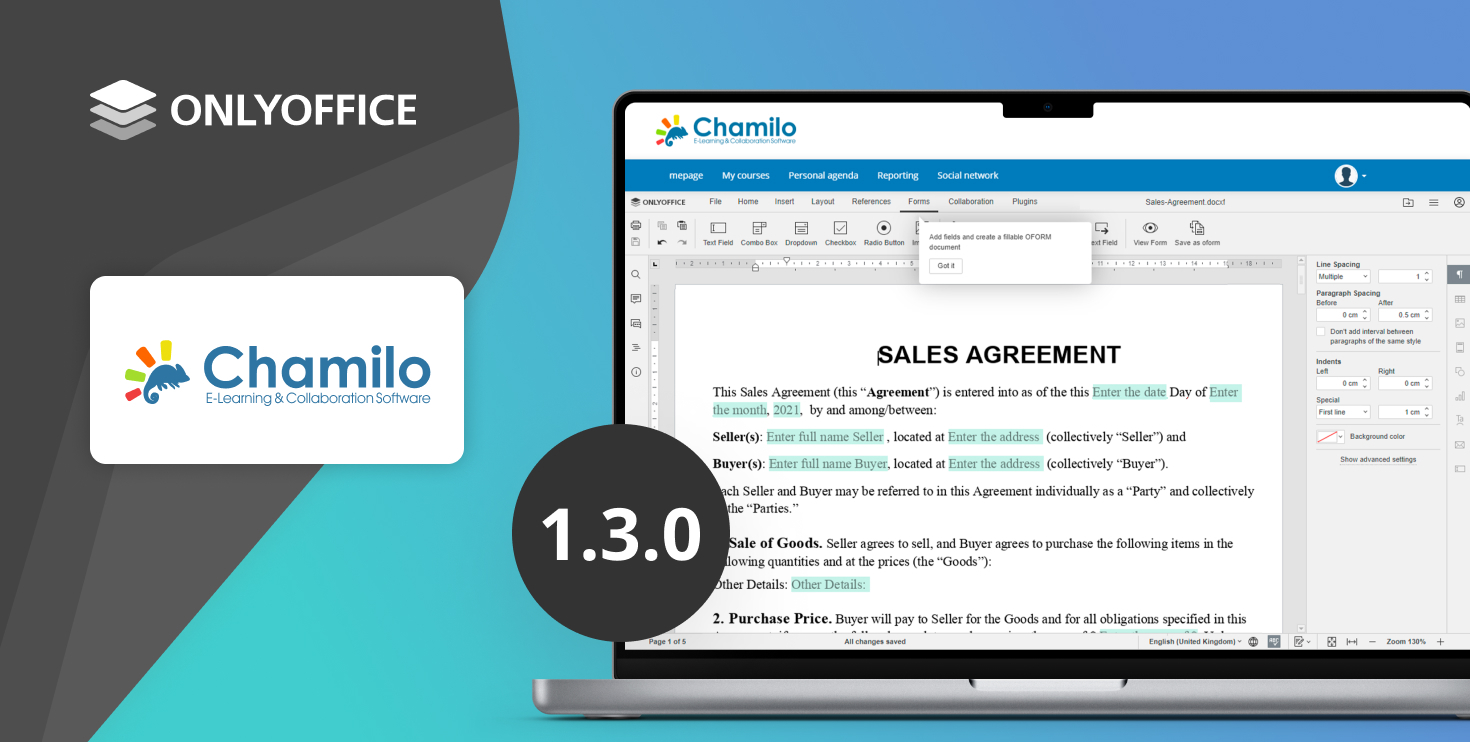# 使用 ONLYOFFICE 宏生成基于性别的个性化问候语

2023日07月19日## 关于性别化

Genderize.io 是一项 API 服务，可预测与名字相关的性别。它为开发人员提供了一种将性别识别功能集成到其应用程序或脚本中的简单方法。通过将名称作为参数发送到 Genderize API，用户会收到包含预测性别和其他信息（例如预测置信度）的响应。Genderize.io 简化了识别名字性别的相关过程。

## 构建宏

``````const oWorksheet = Api.GetActiveSheet()
``````

`````` let ROW = 2
``````

``````function checkRow() {
const name = oWorksheet.GetRange(`A\${ROW}`).GetText()
}
``````

``````   function checkRow() {
const name = oWorksheet.GetRange(`A\${ROW}`).GetText()
if (name === '') {
}
makeRequest(name)
}
``````

makeRequest 函数的目的是将 AJAX 请求发送到 Genderize API：

``````function makeRequest(name) {
\$.ajax({
url: `https://api.genderize.io?name=\${name}`,
dataType: 'json',
}).done(successFunction)
}
``````

``````function successFunction(data) {
if (data.probability > 0.9) {
let title = data.gender === 'male' ? 'Mr' : 'Ms'
}
}
``````

``````function successFunction(data) {
if (data.probability > 0.9) {
let title = data.gender === 'male' ? 'Mr' : 'Ms'
oWorksheet.GetRange(`B\${ROW}`).SetValue(`Dear \${title} \${data.name}!`)
}
ROW++
checkRow()
}
``````

``````    function reload() {
Api.asc_calculate(Asc.c_oAscCalculateType.All);
})
}
})()
``````

``````(function()
{
const oWorksheet = Api.GetActiveSheet()
let ROW = 2
checkRow()

// Recursively request each name until 'A' column value is empty
//
// checkName -> makeRequest -> successFunction (ROW + 1) ->
// checkName -> ... -> checkName -> name is empty -> reload
function checkRow() {
const name = oWorksheet.GetRange(`A\${ROW}`).GetText()
if (name === '') {
}
makeRequest(name)
}

// Request
function makeRequest(name) {
\$.ajax({
url: `https://api.genderize.io?name=\${name}`,
dataType: 'json',
}).done(successFunction)
}
// Response
function successFunction(data) {
if (data.probability > 0.9) {
let title = data.gender === 'male' ? 'Mr' : 'Ms'
oWorksheet.GetRange(`B\${ROW}`).SetValue(`Dear \${title} \${data.name}!`)
}
ROW++
checkRow()
}

// Sheet has to be reloaded on changes
Api.asc_calculate(Asc.c_oAscCalculateType.All);
})
}
})()
``````

### 最新博文## 用于 Chamilo 的 ONLYOFFICE 连接器 v1.3.0：演示服务器、JWT 标头配置、内部请求的地址等更新

2023日11月03日作者: Mona